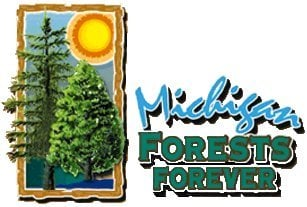Home Page Welcome Page Table of Contents Pre-test Information Tree Basics Section Environment Section Recreation Section Products Section Balance Section Internet Links References Credits Index

MICHIGAN FORESTS FOREVER TEACHERS GUIDE

 TIMBER VOLUME CALCULATION PROCEDUREOverview

Timber volume calculations can be done two ways; 1)  by hand, or 2) using a spreadsheet.  The calculations are the same for both procedures.  Essentially, the process involves the summing of field-collected data and running those sums through a couple of equations.  End results are timber volumes for each species and product (sawtimber and pulpwood), as well as the entire stand (all species) by product.  Basal area also must be calculated, by species and for the entire stand (but not by product).

Both procedures begin with the Field Tally Sheets.  Each field crew has one (maybe two) Field Tally Sheets that contain data collected from all the plots they were able to complete during the field inventory.  Each crew must summarize data on their own Field Tally Sheets, which involves simple math and working with the dot tallies.  This is done by hand.

Once the Field Tally Sheets have been summarized, the sums are run through some simple calculations.  This can be done by hand or by using a spreadsheet.

What to Expect

Some students will have difficulty understanding how to record field data.  Emphasize that EACH DOT (or line) on the Field Tally Sheet represents ONE tree.  There is a tendency to mark a dot or line for each sawlog and pulpstick.  The arrangement of the tables on the Field Tally Sheet make this unnecessary.

Why?  Because the column headings on the tables represent the number of sticks.  A dot below the heading indicates "one tree with that many pulpsticks (or sawlogs).

Some students will have difficulty in following the calculation procedures.  New procedures are often difficult to understand, but this all basic arithmetic.

Calculating Crew Level Tree Counts, Stick Counts, and Sawlog Counts on the Field Tally Sheets

Accurately observed and correctly recorded field data are critical for accuracy (but not likely to happen with students).

The Field Tally Sheet should list all tree species found.  Each species will have its own set of dot tallies.  Sawlogs and pulpsticks were recorded in separate tables.  An example from the pulpstick table . . .

 Species 1 2 3 4 5 6 7 8 #Sticks #Trees Sugar Maple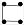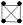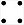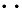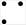To summarize the sugar maple data taken by this crew, the number of pulpsticks in each box must be recorded using numbers.  To do this, multiply the dot tally by the number in the column heading.  Jot that product somewhere in the box.  After the numbers are calculated in each box, add them all together and place that sum in the box on the right labeled "#Sticks".  The number of trees can be counted by simply adding the dot tallies together.  See below.

 Species 1 2 3 4 5 6 7 8 #Sticks #Trees Sugar Maple 73472481524200 67

This procedure needs to be repeated for each species, and then again in the sawlog table.

Calculating Stand Level Volumes and Basal Area

At this point, the Volume Sheet must be used to aggregate the crew data.  This can be done orally and recording numbers on a chalk board, overhead projector, or other method.  These aggregate data are plugged into the volume and basal area equations to calculate stand level information.

List ALL the tree species found by all crews.  For each species, crew totals must be summed for tree count, #pulpsticks, and #sawlogs.  This must be done in two separate tables, one for pulpwood volume and another for sawtimber volume.  Remember that sawtimber tree counts are only for those trees not already counted in the pulpwood tally.  This was part of the field data collection procedures.  Remember to add the grand totals at the bottom of each table where you see the summation symbol (E).

At this point, a choice has to be made about using either a spreadsheet to perform the remaining calculations or to do it by hand.  If you choose to use a spreadsheet, one is available in Microsoft Excel format that can be downloaded.  Stand level volumes and basal area are automatically calculated and can be copied onto the Volume Sheet, or printed.  You can also build your own spreadsheet using the Volume Sheet headings and the calculations below.  If you use the downloaded spreadsheet, you can skip the brown text.

If you have the class calculate volumes by hand, there are now three equations that need to be worked through.  If the calculations are to be done by hand, then the procedure is as follows.  Otherwise, these calculations are built into an Excel spreadsheet that you will have to download.

1.  Pulpwood Volumes

This is an easy calculation, once all the aggregate data have been assembled.  The calculation should be performed for each species and the stand as a whole.

 #sticks + #trees = cords/acre 2 x #plots

2.  Sawtimber Volumes

This calculation is a little more complicated.  First, the average number of logs/tree must be calculated for each species and the stand as a whole (#logs / #trees).  These averages are used to look up a "factor".  Record the averages and factors on the Volume Sheet.  These factors are plugged into the following equation.

 #logs x factor = board feet/acre #plots

3.  Basal Area (square feet/acre)

This has one idiosyncrasy due to simplification of the inventory technique.  Assuming all sawtimber-sized trees have at least one pulpstick above the sawlog portion of the tree, the basal area calculations for just the pulpwood volume would be the same as total stand basal area.  However, once in awhile, a sawtimber-sized tree does not have pulpwood above the sawlog and would not get recorded in the pulpstick tally portion of the Field Tally Sheet.  In most cases, crews will not encounter this situation, meaning the "#trees" column in the Sawlog Tally of the Field Tally Sheet will remain blank, which in turn, means there will be no data in the corresponding table on the Volume Sheet.  Should a tree or two show up in the Sawtimber Volume table on the Volume Sheet, the sawtimber basal area should simply be added to the pulpwood basal area.

Use the following equation to calculate basal area (this only works with a 10-factor tool, such as the penny gauge).

 #tree x 10 = square feet/acre #plotsThis website was developed and created by Michigan State University Extension for the teachers of the State of Michigan.